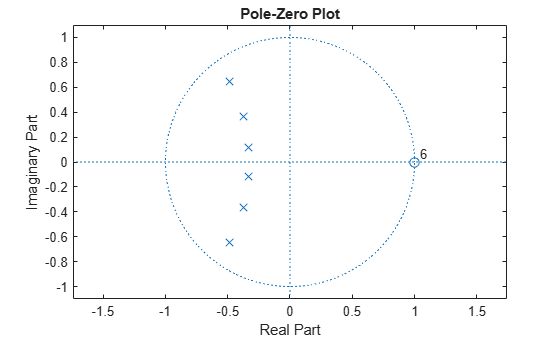# isstable

Determine whether filter is stable

## Syntax

```flag = isstable(b,a) flag = isstable(sos) flag = isstable(d) ```

## Description

`flag = isstable(b,a)` returns a logical output, `flag`, equal to `true` if the filter specified by numerator coefficients, `b`, and denominator coefficients, `a`, is a stable filter. If the poles lie on or outside the circle, `isstable` returns `false`. If the poles are inside the circle, `isstable` returns `true`.

`flag = isstable(sos)` returns `true` if the filter specified by second order sections matrix, `sos`, is stable. `sos` is a K-by-6 matrix, where the number of sections, K, must be greater than or equal to 2. Each row of `sos` corresponds to the coefficients of a second order (biquad) filter. The ith row of the `sos` matrix corresponds to ```[bi(1) bi(2) bi(3) ai(1) ai(2) ai(3)]```.

`flag = isstable(d)` returns `true` if the digital filter, `d`, is stable. Use `designfilt` to generate `d` based on frequency-response specifications.

## Examples

collapse all

Design a sixth-order Butterworth highpass IIR filter using second order sections. Specify a normalized 3-dB frequency of 0.7. Determine if the filter is stable.

```[z,p,k] = butter(6,0.7,'high'); SOS = zp2sos(z,p,k); flag = isstable(SOS) ```
```flag = logical 1 ```
`zplane(z,p)`Redesign the filter using `designfilt` and check it for stability.

```d = designfilt('highpassiir','DesignMethod','butter','FilterOrder',6, ... 'HalfPowerFrequency',0.7); dflg = isstable(d)```
```dflg = logical 1 ```
`zplane(d)`Create a filter and determine its stability at double and single precision.

```b = [1 -0.5]; a = [1 -0.999999999]; act_flag1 = isstable(b,a)```
```act_flag1 = logical 1 ```
`act_flag2 = isstable(single(b),single(a))`
```act_flag2 = logical 0 ```